## Blood Relation – Practice set 14

D.1-3) Study the following information and answer the questions.

P is sister of Q. Q is married to R. R is father of M. S is son of T. Q is mother-in-law of T.

1. R has only one son and no daughter. P is married to K. V is daughter of K.

a) Aunt

b) Brother

c) Sister

d) Mother-in-law

e) Cannot be determined

Click Here To View Answer
a) Aunt

Correct Answer

A

Explanation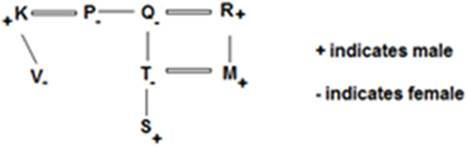2. R has only one son and no daughter. P is married to K. V is daughter of K.

a) Nephew

b) Uncle

c) Brother

d) Grandson

e) Aunt

Click Here To View Answer
d) Grandson

Correct Answer

D

Explanation3. R has only one son and no daughter. P is married to K. V is daughter of K.

a) Niece

b) Sister

c) Cannot be determined

d) Daughter

e) Mother

Click Here To View Answer
a) Niece

Correct Answer

A

Explanation4. A is the son of B, while B and C are sisters to each other. E is the mother of C. If D is the son of E, which of the following statements is correct?

a) D is the maternal uncle of A

b) E is the brother of B

c) D is the cousin of A

d) B and D are brothers.

Click Here To View Answer
a) D is the maternal uncle of A

Correct Answer

A

Explanation

Answer (a)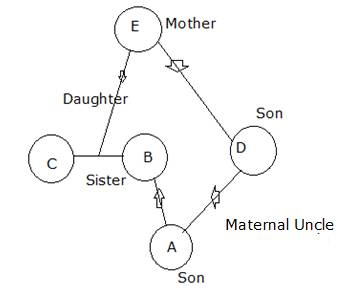5. M is son of P, Q is the grand­daughter of O, who is the husband of P. How is M related to O?

a) Son

b) Daughter

c) Mother

d) Father

Click Here To View Answer
a) Son

Correct Answer

A

Explanation

(a)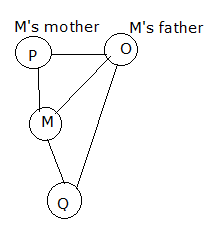6. Pointing to a women, Naman said, “she is the daughter of the only child of my grandmother”. How is the women related to Naman?

a) Niece

b) Cousin

c) Sister

d) None of these

Click Here To View Answer
c) Sister

Correct Answer

C

Explanation

Answer (c)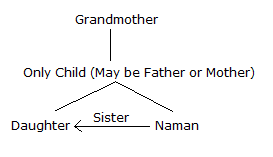7. H is the father of J.J is the brother of L.N is the wife of L.K is the son of N.L and J are children of H.P is the wife of H.K and Z are grandchildren of P. Who is the uncle of K ?

a) J

b) H

c) Z

d) K

e) Cannot be determined

Click Here To View Answer
a) J

Correct Answer

A

8. D is sister of F. M is brother of F. K is brother of D and son of T. R is wife of T. How is F related to T?

a) Son

b) Daughter

c) Son or Daughter

d) Data Inadequate

e) None of these

Click Here To View Answer
c) Son or Daughter

Correct Answer

C

9. Ramu’s mother said to Ramu. “My mother has a son whose son is Achyut”. How is Achyut related to Ramu?

a) Uncle

b) Cousin

c) Brother

d) Nephew

Click Here To View Answer
b) Cousin

Correct Answer

B

Explanation

Answer (b)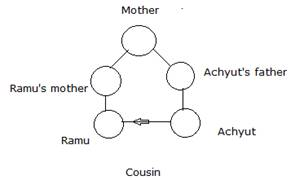10. Introducing Reeta, Monica said, “She is the only daughter of my father’s only daughter.” How is Monica related to Reeta?

a) Aunt

b) Niece

c) Cousin

d) Mother

Click Here To View Answer
d) Mother

Correct Answer

D